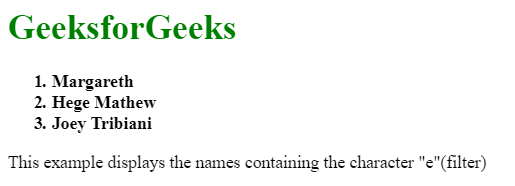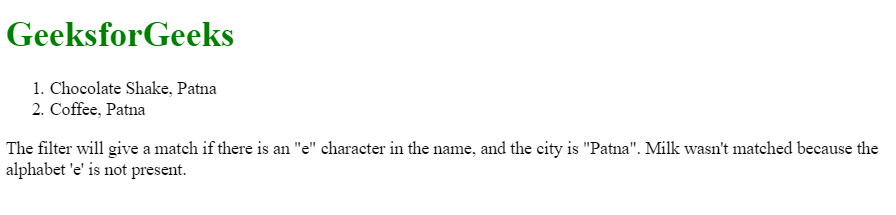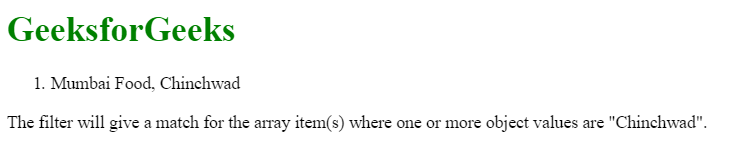# AngularJS | filter Filter

• Last Updated : 17 Jun, 2019

The “filter” Filter in AngularJS is used to filter the array and object elements and return the filtered items. In other words, this filter selects a subset (a smaller array containing elements that meet the filter criteria) of an array from the original array.

Syntax:

```{{ arrayexpression | filter : expression : comparator : anyPropertyKey }}
```

Parameter:

• arrayexpression: The source array on which the filter will be applied.
• expression: It is used to select the items from the array, after the filter conditions are met.
• comparator: It is used to determining the value by comparing the expected value from the filter expression, and the actual value from the object array.
• anyPropertyKey: It is a special property that is used to match the value against given property. Its default value is \$.

Example 1:

 ```<``html``>`` ` `<``head``>``    ``<``title``>AngularJS | filter Filter``     ` `    ``<``script` `src``=``"https://ajax.googleapis.com/ajax/libs/angularjs/1.6.9/angular.min.js"``>``    `````` ` `<``body``>`` ` `    ``<``div` `ng-app``=``"myApp"` `ng-controller``=``"namesCtrl"``>`` ` `        ``<``h1` `style``=``"color:green;"``>GeeksforGeeks`` ` `        ``<``ol``>``            ``<``strong``>``                ``<``li` `ng-repeat``=``"x in names | filter : 'e'"``>``                    ``{{ x }}``                ````            ````        ````    ```` ` `    ``<``script``>``        ``angular.module('myApp', []).controller(``                    ``'namesCtrl', function(\$scope) {``            ``\$scope.names = [``                ``'Jani',``                ``'Carl',``                ``'Margareth',``                ``'Hege Mathew',``                ``'Joey Tribiani',``                ``'Gustav',``                ``'Birgit',``                ``'Mary',``                ``'Kai'``            ``];``        ``});``    ```` ` `    ``<``p``>``        ``This example displays the names``        ``containing the character "e"(filter)``    `````` ` `                    `

Output:Example 2: This example uses an object to filter its element.

 ```<``html``>`` ` `<``head``>``    ``<``title``>AngularJS | filter Filter``     ` `    ``<``script` `src``=``"https://ajax.googleapis.com/ajax/libs/angularjs/1.6.9/angular.min.js"``>``    `````` ` `<``bod``>``    ``<``div` `ng-app``=``"myApp"` `ng-controller``=``"arrCtrl"``>``  ` `        ``<``h1` `style``=``"color:green"``>GeeksforGeeks``  ` `        ``<``ol``>``            ``<``li` `ng-repeat``=``            ``"x in customers | filter :{'name' : 'e', 'city' : 'Patna'}"``>``                ``{{x.name + ", " + x.city}}``            ````        ````    ````     ` `  ` `    ``<``script``>``        ``var app = angular.module('myApp', []);``     ` `        ``app.controller('arrCtrl', function(\$scope) {``            ``\$scope.customers = [{``                ``"name": "Chocolate Shake",``                ``"city": "Patna"``            ``}, {``                ``"name": "Hot Chocolate",``                ``"city": "Delhi"``            ``}, {``                ``"name": "Milk",``                ``"city": "Patna"``            ``}, {``                ``"name": "Coffee",``                ``"city": "Patna"``            ``}, {``                ``"name": "Tea",``                ``"city": "Pune"``            ``}, {``                ``"name": "Mineral Water",``                ``"city": "Mumbai"``            ``}, {``                ``"name": "Iced Tea",``                ``"city": "Bangalore"``            ``}];``        ``});``    ````  ` `    ``<``p``>``        ``The filter will give a match if there is``        ``an "e" character in the name, and the city``        ``is "Patna". Milk wasn't matched because``        ``the alphabet 'e' is not present.``    ```` ` ```                    `

Output:Example 3: In this example, we are going to see a “strict” caparison which does not return any value unless it is a pure match.

 ```<``html``>`` ` `<``head``>``    ``<``title``>AngularJS | filter Filter``     ` `    ``<``script` `src``=``"https://ajax.googleapis.com/ajax/libs/angularjs/1.6.9/angular.min.js"``>``    `````` ` `<``body``>``  ` `    ``<``div` `ng-app``=``"myApp"` `ng-controller``=``"arrCtrl"``>``        ``<``h1` `style``=``"color:green"``>GeeksforGeeks``         ` `        ``<``ol``>``            ``<``li` `ng-repeat``=``"x in customers | filter : 'Chinchwad' : true"``>``              ``{{x.name + ", " + x.city}}``            ````        ````    ````  ` `    ``<``script``>``        ``var app = angular.module('myApp', []);``         ` `        ``app.controller('arrCtrl', function(\$scope) {``            ``\$scope.customers = [{``                    ``"name": "Mumbai Food",``                    ``"city": "Chinchwad"``                ``}, {``                    ``"name": "Gurgaon Catering",``                    ``"city": "Haryana City"``                ``}, {``                    ``"name": "Noida temperature",``                    ``"city": " Chinchwad"``                ``},``  ` `            ``];``        ``});``    ````  ` `    ``<``p``>The filter will give a match for the array item(s) ``       ``where one or more object values are "Chinchwad".``  ` ```                    `

Output:Note: If you notice that the last Chinchwad in the array is not included in the list, because the last Chinchwad containing a space before itself.

My Personal Notes arrow_drop_up